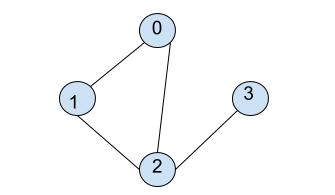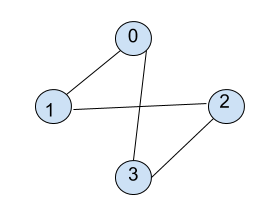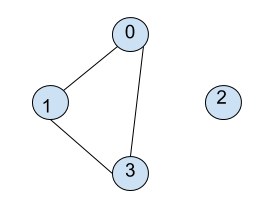New update is available. Click here to update.

# BFS in Graph

Contributed by
Arindam Majumder
Last Updated: 23 Feb, 2023
Easy0/40
Avg time to solve 10 mins
Success Rate 90 %Share80 upvotes

## Problem Statement

#### Note:

``````1. Here, you need to consider that you need to print the BFS path starting from vertex 0 only.
2. V is the number of vertices present in graph G, and all vertices are numbered from 0 to V-1.
3. E is the number of edges present in graph G.
4. Graph input is provided as the number of vertices and a list of edges.
5. Handle for Disconnected Graphs as well.
``````

#### For Example: Consider graph:``````Here, starting from 0, it is connected to 1 and 2 so, BFS traversal from here will be [0, 1, 2 ]. Now, 3 is also connected to 2. So, BFS traversal becomes [0, 1, 2, 3].
``````

#### Note:

``````For each node, the correct order of printing the connected nodes will be sorted order, i.e., if {3,6,9,4} are connected to 1, then the correct order of their printing is {1,3,4,6,9}.
``````
Detailed explanation ( Input/output format, Notes, Images )##### Sample Input 1:
``````4 4
0 1
0 3
1 2
2 3
``````
##### Sample Output 1:
``````0 1 3 2
``````
##### Explanation for sample input 1:``````Starting from 0, it is connected to 1 and 3, which will be printed. Then comes 2, which was connected to 1.
``````
##### Sample Input 2:
``````4 3
0 1
0 3
1 3
``````
##### Sample Output 2:
``````0 1 3 2
``````
##### Explanation for sample input 2:``````Starting from 0, it is connected to 1 and 3, which will be printed. The remaining node is 2, which will be printed at the end.
``````AutoConsole